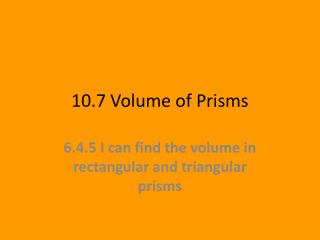# 10.7 Volume of Prisms - PowerPoint PPT PresentationDownload Presentation10.7 Volume of Prisms

10.7 Volume of Prisms
Download Presentation## 10.7 Volume of Prisms

- - - - - - - - - - - - - - - - - - - - - - - - - - - E N D - - - - - - - - - - - - - - - - - - - - - - - - - - -
##### Presentation Transcript

1. 10.7 Volume of Prisms 6.4.5 I can find the volume in rectangular and triangular prisms

2. Warm Up Find the area of each figure. Use 3.14 for . 1. rectangle with base length 8 in. and height 12 in. 96 in2 2. circle with diameter 8 ft 50.24 ft2

3. Volume is the number of cubic units needed to fill a space. To find the volume of prisms you simply multiply the area of the base times the height. V = area of base x height

4. To find the volume of any prism, you can use the formula V= Bh, where B is the area of the base, and h is the prism’s height.

5. V = 3,718 in3

6. 16 in. 12 in. 29 in. V = 5,568 in3

7. V = 10.14 m3

8. V = 136.5 ft3 Find the volume of the triangular prism.

9. V = Bh V = 23.52 m3 7 m 1.6 m 4.2 m

10. 3,600 cm3 153.3 cm3 38.13 cm3 Find the volume of each figure. 1. rectangular prism with length 20 cm, width 15 cm, and height 12 cm 2. triangular prism with a height of 12 cm and a triangular base with base length 7.3 cm and height 3.5 cm 3. Find the volume of the figure shown.

11. Identify the volume of a rectangular prism with length 25 cm, width 20 cm, and height 10 cm. A. 5,000 cm3 B. 5,225 cm3 C. 5,450 cm3 D. 5,650 cm3

12. Identify the volume of the figure shown. A. 72.52 cm3 B. 63.64 cm3 C. 62.5 cm3 D. 61.67 cm3

13. 10.8 Volume of Cylinders 6.4.5 I can find the volumes of cylinders

14. 1,320 cm3 359.04 cm3 Warm Up Find the volume of each figure described. 1. rectangular prism with length 12 cm, width 11 cm, and height 10 cm 2. triangular prism with height 11 cm and triangular base with base length 10.2 cm and height 6.4 cm

15. To find the volume of a cylinder, you can use the same method as you did for prisms: Multiply the area of the base by the height. volume of a cylinder = area of base  height The area of the circular base is r2, so the formula is V = Bh = r2h.

16. V 351.68 The volume is about 352 ft3. Find the volume V of the cylinder to the nearest cubic unit. V = r2h

17. V 863.5 The volume is about 864 cm3. Find the volume V of the cylinder to the nearest cubic unit.

18. V 565.2 The volume is about 565 ft3. Find the volume V of the cylinder to the nearest cubic unit. 6 ft 5 ft

19. V 301.44 The volume is about 301 cm3. 8 cm 6 cm

20. V 1,230.88 The volume is about 1,231 in3. h r = + 5 4 h = 8 in

21. V 1,384.74 The volume is about 1,385 in3.

22. V 84.78 cm3 V 169.56 cm3 Cylinder 2 has the greater volume because 169.56 cm3 > 84.78 cm3. Find which cylinder has the greater volume. Cylinder 1: Cylinder 2:

23. 10 cm 2.5 cm V 196.25 cm3 4 cm 4 cm V 50.24 cm3 Cylinder 1 has the greater volume because 196.25 cm3 > 50.24 cm3. Find which cylinder has the greater volume. Cylinder 1: Cylinder 2:

24. Lesson Quiz for Student Response Systems 1. Identify the volume of a cylinder with a radius of 11 ft and a height of 5 ft to the nearest cubic unit. Use 3.14 for . A. 1,900 ft3 B. 1,890 ft3 C. 1,706 ft3 D. 690 ft3

25. Lesson Quiz for Student Response Systems 2. Identify the volume of a cylinder with a radius of 4.3 ft and a height of 5 ft to the nearest cubic unit. Use 3.14 for . A. 338 ft3 B. 305 ft3 C. 297 ft3 D. 290 ft3